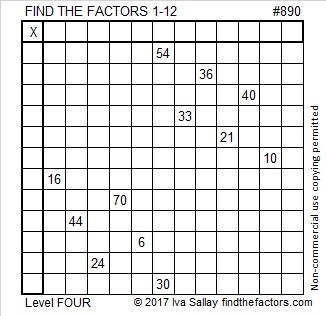# 890 and Level 4

890 is the sum of four consecutive prime numbers:

• 211 + 223 + 227 + 229 = 890

890 is also the hypotenuse of four Pythagorean triples:

• 168-874-890, which is 2 times (84-437-445)
• 390-800-890, which is 10 times (39-80-89)
• 406-792-890, which is 2 times (203-396-445)
• 534-712-890, which is (3-4-5) times 178Print the puzzles or type the solution on this excel file: 12 factors 886-896

• 890 is a composite number.
• Prime factorization: 890 = 2 × 5 × 89
• The exponents in the prime factorization are 1, 1, and 1. Adding one to each and multiplying we get (1 + 1)(1 + 1)(1 + 1) = 2 × 2 × 2 = 8. Therefore 890 has exactly 8 factors.
• Factors of 890: 1, 2, 5, 10, 89, 178, 445, 890
• Factor pairs: 890 = 1 × 890, 2 × 445, 5 × 178, or 10 × 89
• 890 has no square factors that allow its square root to be simplified. √890 ≈ 29.83286778.This site uses Akismet to reduce spam. Learn how your comment data is processed.